|

# 扮靓你的卫浴间 导购大全之各风格浴室柜推荐

在卫浴间中，能够与人产生情感对话的往往有两种产品，分别是浴缸和浴室柜。躺在浴缸里面沐浴，就是一种对话，感觉根据不同人的思想而不同，然而由于生活节奏的愈加快速，大大限制了我们与浴缸的交流时间。浴室柜，则大为不一样，每人每天的早晨与晚上都要与它进行全方位的对话，手摸龙头是一种触感、眼看镜子是一种视觉，水洗在面上则是一种体验。正因为如此，浴室柜的实用功能、外观设计等都会直接影响一个人的心情。

所以随着人们对这种情感需求的强烈，风格多样的精巧的浴室柜也逐渐吸引了大家的视线，越来越多的色彩和款式成为卫浴间的视觉亮点。今天小编就给大家汇总了三种风格的浴室柜，让渴望在浴室空间和自己对话的你，有个更好的体验。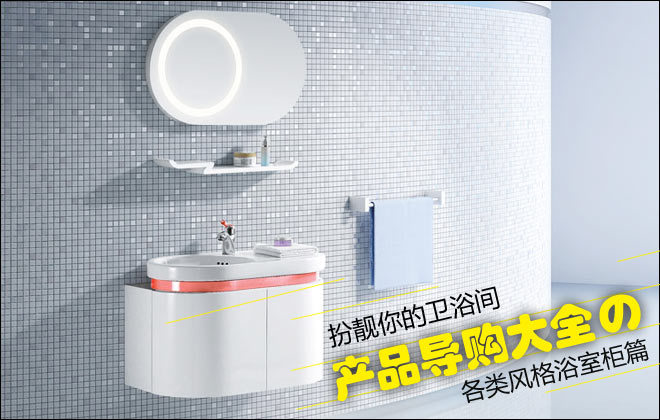风格1：清新风格

清新简约风格的浴室柜，少在柜面的设计上，添加华而不实的装饰细节。在表现上，如日式现代风格中，比较注重表现柜面的原木颜色与质感。有些则直接用上PVC等现代材料作为柜体的设计。这种风格的浴室柜非常适合小户型卫浴风格的装修。

推荐产品1：箭牌APG8G341L浴室柜

参考价格：1368元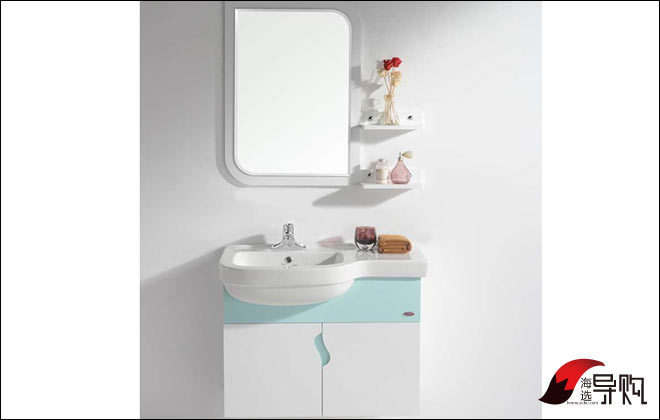箭牌APG8G341L浴室柜清新亮丽的外观给你家的浴室柜注入了不一样的时尚元素，同样以白色为主色调，给人一种清新自然的感觉。采用PVC材质，耐潮耐磨而且非常的环保，整个将现代家居的时尚简约发挥的淋漓尽致。优美的曲线加上自然的色调，非常适合追求简约生活的现代人使用。

推荐产品2：心海伽蓝实木卫浴柜

参考价格：1099元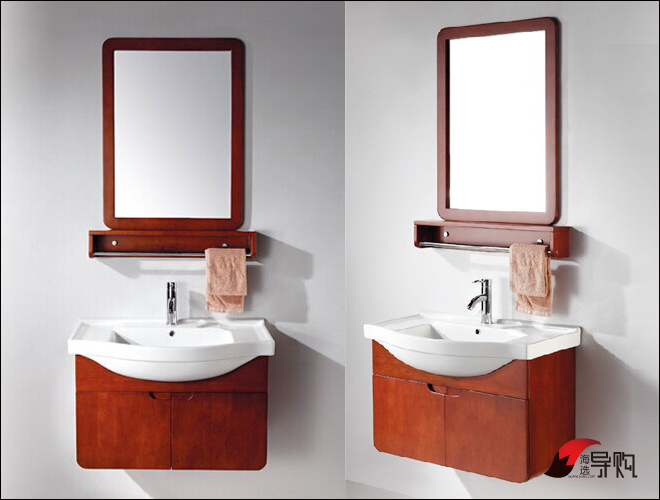心海伽蓝实木卫浴柜采用四周圆角设计，保护家人特别是小孩不会磕碰受伤，比较人性化。采用东南亚进口橡胶木制作，符合国际环保标准要求，它拥有120mm的托架宽度，满足您日常生活用品的放置，可摆放一些化妆品，洗漱品等。大肚盆造型的陶瓷盆，储水空间非常充裕，方便洗刷东西。还有人性化挡水沿设计，防止洗涤时水流溢出。

更多家居导购===========================

【海选】玄关怎么装？三招彻底秒杀脏乱差>>

【海选】花好月圆情意浓 5款精致餐具推荐>>

【海选】瓷砖环保谁说了算 符合3C认证抛光砖推荐>>

【海选】摆脱平民淋浴形象 导购大全之功能花洒篇>>X

在卫浴间中，能够与人产生情感对话的往往有两种产品，分别是浴缸和浴室柜。躺在浴缸里面沐浴，就是一种对话，感觉根据不同人的思想而不同，然而由于生活节奏的愈加快速，大大限制了我们与浴缸的交流时间。浴室柜，则大为不一样，每人每天的早晨与晚上都要与它进行全方位的对话，手摸龙头是一种触感、眼看镜子是一种视觉，水洗在面上则是一种体验。正因为如此，浴室柜的实用功能、外观设计等都会直接影响一个人的心情。

所以随着人们对这种情感需求的强烈，风格多样的精巧的浴室柜也逐渐吸引了大家的视线，越来越多的色彩和款式成为卫浴间的视觉亮点。今天小编就给大家汇总了三种风格的浴室柜，让渴望在浴室空间和自己对话的你，有个更好的体验。

风格2：中式风格

现在中式风格浴室柜也越来越受到欢迎。其实对于选择中式风格的人来说，“浴室柜选择什么风格”可能根本就不是问题，因为选择中式风格的人一般都对它情有独钟，一方面它保留了其原材质、色彩的大致风格，另一方面又体现出传统的历史痕迹和浑厚的文化底蕴，同时还没有过于复杂的装饰，简化了浴室柜本身的线条。配合中式家居，体现出主人或儒雅、或精致、或平和的气质。

推荐产品1：箭牌APGM348实木浴室柜

参考价格：3280元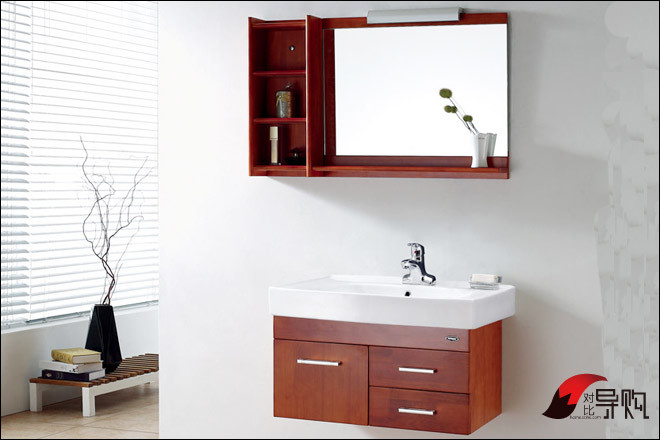箭牌APGM348实木浴室柜，其桔红色的外表顿时给人带来强烈的视觉冲击，外观造型略显方正，端庄稳健。浴盆边缘呈弧线型设计，时尚美观，至于浴室当中，能为家居生活带来轻松愉悦之感。柜式的结构，不仅节省了卫浴空间，而且也大大提高这款浴室柜的实用性。

推荐产品2：惠达HDFL155_03浴室柜

参考价格：3190元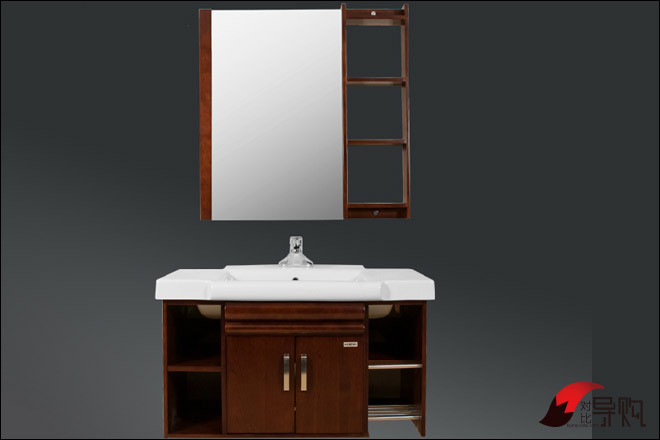惠达HDFL155_03浴室柜产品整体造型简单，外观比较大气，实木色彩更加时尚，比较具有档次。其材质是采用多层实木板，不仅环保，而且淋漓尽致体现了中式风格浴室柜的大气。

风格3：欧式古典风格

欧式风格浴室柜主要是运用了超越现实和豪华装饰手法，开放、创新的设计思想以及尊贵的姿容。这类的浴室柜适合稍大型的卫生间，特别是别墅。配合家的大气与雍和，你甚至可以从卫生间的细节感受到贵族般的严谨。

推荐产品：东鹏JG0011231Q浴室柜

参考价格：8600元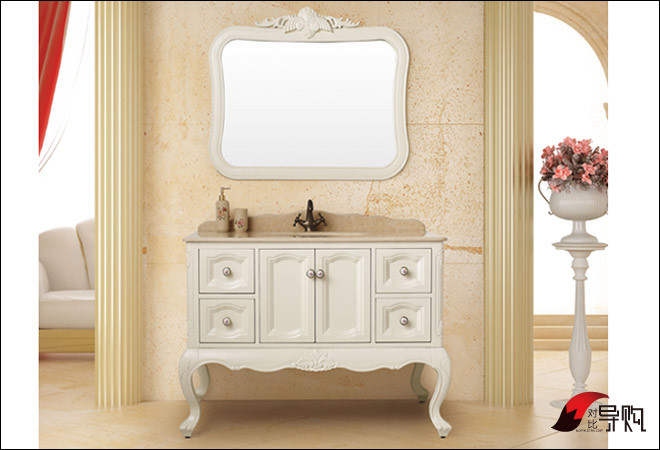东鹏JG0011231Q欧式浴室柜为仿古设计，整体以欧式经典风格为主，天然大理石台面再现尊贵，是当下设计师们，而且还融入了中国的“虎腿”设计，追求创新突破的"混搭"典范。色调以白色为基调，优雅纯美，时尚贵气。整个浴室柜庄重大气，尽显欧式贵族风范。拉手的经典欧式雕花为整个浴室柜增添了一丝浪漫气质，实为点睛之笔。

编辑点评：各个风格的浴室柜都有其适合的人群，简约风格比较适合小户型也比较适合年轻人；中式风格则适合上了年纪而且对卫浴产品有要求的中年人；欧式风格换小编的话来说，就是比较适合生活富足的土豪们了，因为它的大气足以撑起大户型的装修。想要购买浴室柜的朋友，不妨对号入座，选择自己所喜欢风格的产品吧。

`声明：本文由入驻焦点开放平台的作者撰写，除焦点官方账号外，观点仅代表作者本人，不代表焦点立场错误信息举报电话： 400-099-0099，邮箱：jubao@vip.sohu.com，或点此进行意见反馈，或点此进行举报投诉。`A B C D E F G H J K L M N P Q R S T W X Y Z
A - B - C - D - E
• A
• 鞍山
• 安庆
• 安阳
• 安顺
• 安康
• 澳门
• B
• 北京
• 保定
• 包头
• 巴彦淖尔
• 本溪
• 蚌埠
• 亳州
• 滨州
• 北海
• 百色
• 巴中
• 毕节
• 保山
• 宝鸡
• 白银
• 巴州
• C
• 承德
• 沧州
• 长治
• 赤峰
• 朝阳
• 长春
• 常州
• 滁州
• 池州
• 长沙
• 常德
• 郴州
• 潮州
• 崇左
• 重庆
• 成都
• 楚雄
• 昌都
• 慈溪
• 常熟
• D
• 大同
• 大连
• 丹东
• 大庆
• 东营
• 德州
• 东莞
• 德阳
• 达州
• 大理
• 德宏
• 定西
• 儋州
• 东平
• E
• 鄂尔多斯
• 鄂州
• 恩施
F - G - H - I - J
• F
• 抚顺
• 阜新
• 阜阳
• 福州
• 抚州
• 佛山
• 防城港
• G
• 赣州
• 广州
• 桂林
• 贵港
• 广元
• 广安
• 贵阳
• 固原
• H
• 邯郸
• 衡水
• 呼和浩特
• 呼伦贝尔
• 葫芦岛
• 哈尔滨
• 黑河
• 淮安
• 杭州
• 湖州
• 合肥
• 淮南
• 淮北
• 黄山
• 菏泽
• 鹤壁
• 黄石
• 黄冈
• 衡阳
• 怀化
• 惠州
• 河源
• 贺州
• 河池
• 海口
• 红河
• 汉中
• 海东
• 怀来
• I
• J
• 晋中
• 锦州
• 吉林
• 鸡西
• 佳木斯
• 嘉兴
• 金华
• 景德镇
• 九江
• 吉安
• 济南
• 济宁
• 焦作
• 荆门
• 荆州
• 江门
• 揭阳
• 金昌
• 酒泉
• 嘉峪关
K - L - M - N - P
• K
• 开封
• 昆明
• 昆山
• L
• 廊坊
• 临汾
• 辽阳
• 连云港
• 丽水
• 六安
• 龙岩
• 莱芜
• 临沂
• 聊城
• 洛阳
• 漯河
• 娄底
• 柳州
• 来宾
• 泸州
• 乐山
• 六盘水
• 丽江
• 临沧
• 拉萨
• 林芝
• 兰州
• 陇南
• M
• 牡丹江
• 马鞍山
• 茂名
• 梅州
• 绵阳
• 眉山
• N
• 南京
• 南通
• 宁波
• 南平
• 宁德
• 南昌
• 南阳
• 南宁
• 内江
• 南充
• P
• 盘锦
• 莆田
• 平顶山
• 濮阳
• 攀枝花
• 普洱
• 平凉
Q - R - S - T - W
• Q
• 秦皇岛
• 齐齐哈尔
• 衢州
• 泉州
• 青岛
• 清远
• 钦州
• 黔南
• 曲靖
• 庆阳
• R
• 日照
• 日喀则
• S
• 石家庄
• 沈阳
• 双鸭山
• 绥化
• 上海
• 苏州
• 宿迁
• 绍兴
• 宿州
• 三明
• 上饶
• 三门峡
• 商丘
• 十堰
• 随州
• 邵阳
• 韶关
• 深圳
• 汕头
• 汕尾
• 三亚
• 三沙
• 遂宁
• 山南
• 商洛
• 石嘴山
• T
• 天津
• 唐山
• 太原
• 通辽
• 铁岭
• 泰州
• 台州
• 铜陵
• 泰安
• 铜仁
• 铜川
• 天水
• 天门
• W
• 乌海
• 乌兰察布
• 无锡
• 温州
• 芜湖
• 潍坊
• 威海
• 武汉
• 梧州
• 渭南
• 武威
• 吴忠
• 乌鲁木齐
X - Y - Z
• X
• 邢台
• 徐州
• 宣城
• 厦门
• 新乡
• 许昌
• 信阳
• 襄阳
• 孝感
• 咸宁
• 湘潭
• 湘西
• 西双版纳
• 西安
• 咸阳
• 西宁
• 仙桃
• 西昌
• Y
• 运城
• 营口
• 盐城
• 扬州
• 鹰潭
• 宜春
• 烟台
• 宜昌
• 岳阳
• 益阳
• 永州
• 阳江
• 云浮
• 玉林
• 宜宾
• 雅安
• 玉溪
• 延安
• 榆林
• 银川
• Z
• 张家口
• 镇江
• 舟山
• 漳州
• 淄博
• 枣庄
• 郑州
• 周口
• 驻马店
• 株洲
• 张家界
• 珠海
• 湛江
• 肇庆
• 中山
• 自贡
• 资阳
• 遵义
• 昭通
• 张掖
• 中卫

1室1厅1厨1卫1阳台

1
2
3
4
5

0
1
2

1

1

0
1
2
3报名成功，资料已提交审核A B C D E F G H J K L M N P Q R S T W X Y Z
A - B - C - D - E
• A
• 鞍山
• 安庆
• 安阳
• 安顺
• 安康
• 澳门
• B
• 北京
• 保定
• 包头
• 巴彦淖尔
• 本溪
• 蚌埠
• 亳州
• 滨州
• 北海
• 百色
• 巴中
• 毕节
• 保山
• 宝鸡
• 白银
• 巴州
• C
• 承德
• 沧州
• 长治
• 赤峰
• 朝阳
• 长春
• 常州
• 滁州
• 池州
• 长沙
• 常德
• 郴州
• 潮州
• 崇左
• 重庆
• 成都
• 楚雄
• 昌都
• 慈溪
• 常熟
• D
• 大同
• 大连
• 丹东
• 大庆
• 东营
• 德州
• 东莞
• 德阳
• 达州
• 大理
• 德宏
• 定西
• 儋州
• 东平
• E
• 鄂尔多斯
• 鄂州
• 恩施
F - G - H - I - J
• F
• 抚顺
• 阜新
• 阜阳
• 福州
• 抚州
• 佛山
• 防城港
• G
• 赣州
• 广州
• 桂林
• 贵港
• 广元
• 广安
• 贵阳
• 固原
• H
• 邯郸
• 衡水
• 呼和浩特
• 呼伦贝尔
• 葫芦岛
• 哈尔滨
• 黑河
• 淮安
• 杭州
• 湖州
• 合肥
• 淮南
• 淮北
• 黄山
• 菏泽
• 鹤壁
• 黄石
• 黄冈
• 衡阳
• 怀化
• 惠州
• 河源
• 贺州
• 河池
• 海口
• 红河
• 汉中
• 海东
• 怀来
• I
• J
• 晋中
• 锦州
• 吉林
• 鸡西
• 佳木斯
• 嘉兴
• 金华
• 景德镇
• 九江
• 吉安
• 济南
• 济宁
• 焦作
• 荆门
• 荆州
• 江门
• 揭阳
• 金昌
• 酒泉
• 嘉峪关
K - L - M - N - P
• K
• 开封
• 昆明
• 昆山
• L
• 廊坊
• 临汾
• 辽阳
• 连云港
• 丽水
• 六安
• 龙岩
• 莱芜
• 临沂
• 聊城
• 洛阳
• 漯河
• 娄底
• 柳州
• 来宾
• 泸州
• 乐山
• 六盘水
• 丽江
• 临沧
• 拉萨
• 林芝
• 兰州
• 陇南
• M
• 牡丹江
• 马鞍山
• 茂名
• 梅州
• 绵阳
• 眉山
• N
• 南京
• 南通
• 宁波
• 南平
• 宁德
• 南昌
• 南阳
• 南宁
• 内江
• 南充
• P
• 盘锦
• 莆田
• 平顶山
• 濮阳
• 攀枝花
• 普洱
• 平凉
Q - R - S - T - W
• Q
• 秦皇岛
• 齐齐哈尔
• 衢州
• 泉州
• 青岛
• 清远
• 钦州
• 黔南
• 曲靖
• 庆阳
• R
• 日照
• 日喀则
• S
• 石家庄
• 沈阳
• 双鸭山
• 绥化
• 上海
• 苏州
• 宿迁
• 绍兴
• 宿州
• 三明
• 上饶
• 三门峡
• 商丘
• 十堰
• 随州
• 邵阳
• 韶关
• 深圳
• 汕头
• 汕尾
• 三亚
• 三沙
• 遂宁
• 山南
• 商洛
• 石嘴山
• T
• 天津
• 唐山
• 太原
• 通辽
• 铁岭
• 泰州
• 台州
• 铜陵
• 泰安
• 铜仁
• 铜川
• 天水
• 天门
• W
• 乌海
• 乌兰察布
• 无锡
• 温州
• 芜湖
• 潍坊
• 威海
• 武汉
• 梧州
• 渭南
• 武威
• 吴忠
• 乌鲁木齐
X - Y - Z
• X
• 邢台
• 徐州
• 宣城
• 厦门
• 新乡
• 许昌
• 信阳
• 襄阳
• 孝感
• 咸宁
• 湘潭
• 湘西
• 西双版纳
• 西安
• 咸阳
• 西宁
• 仙桃
• 西昌
• Y
• 运城
• 营口
• 盐城
• 扬州
• 鹰潭
• 宜春
• 烟台
• 宜昌
• 岳阳
• 益阳
• 永州
• 阳江
• 云浮
• 玉林
• 宜宾
• 雅安
• 玉溪
• 延安
• 榆林
• 银川
• Z
• 张家口
• 镇江
• 舟山
• 漳州
• 淄博
• 枣庄
• 郑州
• 周口
• 驻马店
• 株洲
• 张家界
• 珠海
• 湛江
• 肇庆
• 中山
• 自贡
• 资阳
• 遵义
• 昭通
• 张掖
• 中卫• 手机• 分享
• 设计
免费设计
• 计算器
装修计算器
• 入驻
合作入驻
• 联系
联系我们
• 置顶
返回顶部